Home > CCA2 > Chapter 7 > Lesson 7.2.4 > Problem7-161

7-161.
1. Recall the strategies you developed for converting degrees to radians. How could you reverse that? Convert each of the following angle measures. Be sure to show all of your work. Homework Help ✎

1. π radians to degrees

2. 3π radians to degrees

3. 30 degrees to radians

4.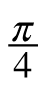radians to degrees

5. 225 degrees to radians

6.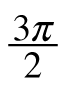radians to degrees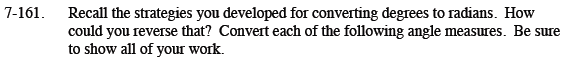Recall that π radians are equal to 180º.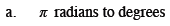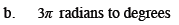$30^\circ\left( \frac{\pi}{180^\circ} \right)$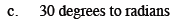$\left( \frac{\pi}{4} \right) \left( \frac{180^\circ}{\pi} \right)$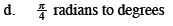$\frac{5\pi}{4} \; \text{radians}$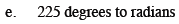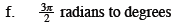270°